# High School Math : Right Triangles

## Example Questions

### Example Question #8 : Triangles

If the hypotenuse of a triangle is 5 meters, which of the following is the closest value to the area of the triangle?

5

54

12

45

26

12

Explanation:

The answer is 12. In this circumstance, the area of the triangle cannot be smaller than its hypotenuse length, and cannot be bigger than its hypotenuse squared (that would be the area of a square).

### Example Question #9 : Triangles

Triangle ABC is drawn between the points A(4, 3), B(4, 8), and C(7, 3). What is the area of ABC?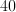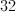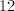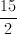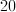Explanation:

Drawing a quick sketch of this triangle will reveal that it is a right triangle. The lines AB and AC form the height and base of this triangle interchangeably, depending on how you look at it.

Either way the formula for the area of the triangle is the distance from A to B multiplied by the distance from A to C, divided by 2.

This is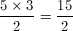### Example Question #2 : How To Find The Area Of A Right Triangle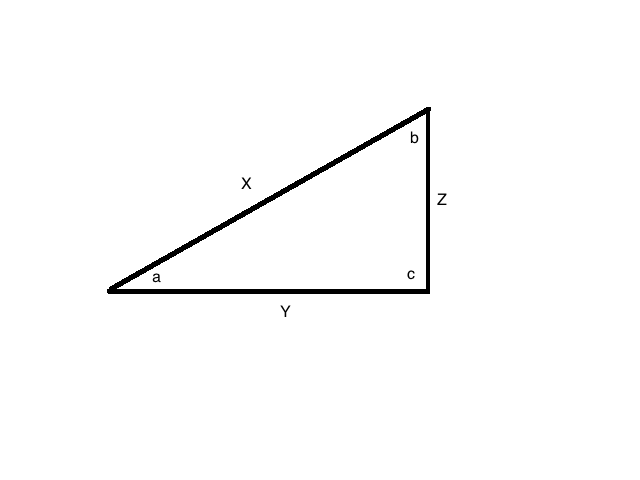If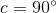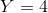, and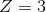,  what is the area of this triangle?Explanation:

The formula for area of a triangble is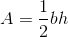.

Since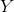will be the base and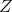will be the height.

Plug in our given values to solve: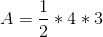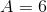### Example Question #3 : How To Find The Area Of A Right Triangle

Find the area of the following triangle: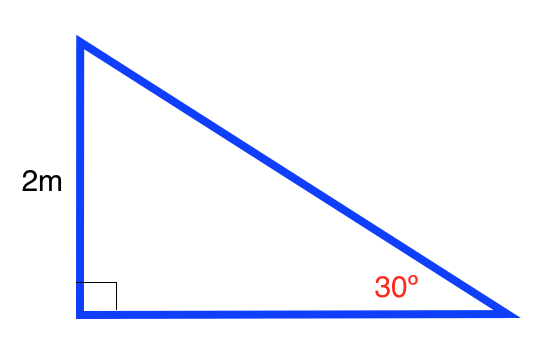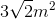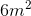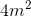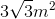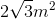Explanation:

The formula for the area of a right triangle is: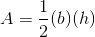whereis the length of the base andis the length of the height.

Use the formulas for a a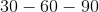triangle to find the length of the base. The formula for atriangle is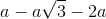.

Ourtriangle is: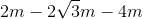Plugging in our values, we get: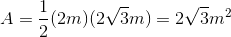### Example Question #4 : How To Find The Area Of A Right Triangle

Find the area of the following right triangle: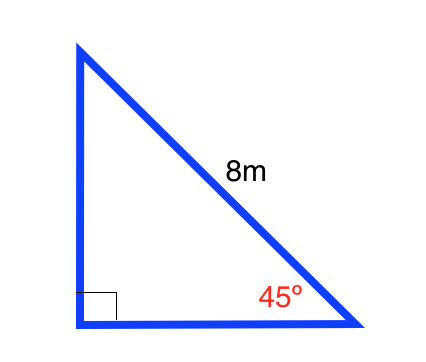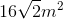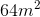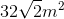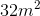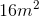Explanation:

The formula for the area of a right triangle is:whereis the length of the base andis the length of the height.

Use the formulas for a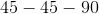triangle to find the length of the base and height. The formula for atriangle is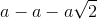Ourtriangle is: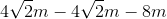Plugging in our values, we get: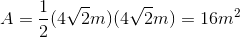### Example Question #5 : How To Find The Area Of A Right Triangle

You are given a right triangle with legs of lengthfeet andfeet. What is the area of this triangle?square feetsquare feetsquare feetsquare feetsquare feetsquare feet

Explanation:

Since we have a right triangle, and are given the lengths of the legs, we are by definition given the base and height. Recall that the legs of the triangle are those next to the right angle, and the hypotenuse is across from the right angle.

Recall the formula for the area of a triangle: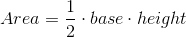Since we know the base and height we plug them into the equation above: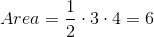We are given units of feet, so the area will be in terms of square feet. Then, our answer issquare feet.

### Example Question #10 : Right Triangles

The ratio for the side lengths of a right triangle is 3:4:5. If the perimeter is 48, what is the area of the triangle?

96

108

50

48

240

96

Explanation:

We can model the side lengths of the triangle as 3x, 4x, and 5x. We know that perimeter is 3x+4x+5x=48, which implies that x=4. This tells us that the legs of the right triangle are 3x=12 and 4x=16, therefore the area is A=1/2 bh=(1/2)(12)(16)=96.

### Example Question #61 : Plane Geometry

The length of one leg of an equilateral triangle is 6. What is the area of the triangle?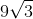Explanation: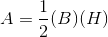The base is equal to 6.

The height of an quilateral triangle is equal to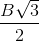, whereis the length of the base.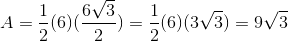### Example Question #1 : Triangles

Acute angles x and y are inside a right triangle. If x is four less than one third of 21, what is y?

7

87

90

3

18

87

Explanation:

We know that the sum of all the angles must be 180 and we already know one angle is 90, leaving the sum of x and y to be 90.

Solve for x to find y.

One third of 21 is 7. Four less than  7 is 3. So if angle x is 3 then that leaves 87 for angle y.

### Example Question #2 : Triangles

If a right triangle has one leg with a length of 4 and a hypotenuse with a length of 8, what is the measure of the angle between the hypotenuse and its other leg?

60

65

90

45

30